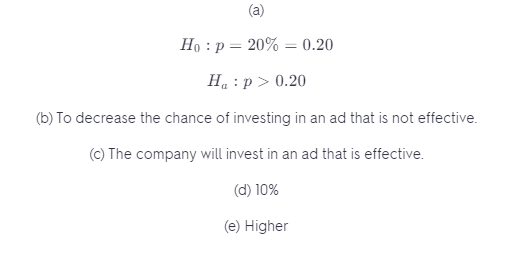# A Company Is Willing To Renew Its Advertising

We thoroughly check each answer to a question to provide you with the most correct answers. Found a mistake? Let us know about it through the REPORT button at the bottom of the page.

A company is willing to renew its advertising contract with a local radio station only if the station can prove that more than 20% of the residents of the city have heard the ad and recognize the company’s product. The radio station conducts a random phone survey of 400 people.

a) What are the hypotheses?

b) The station plans to conduct this test using a 10% level of significance, but the company wants the significance level lowered to 5%. Why?

c) What is meant by the power of this test?

d) For which level of significance will the power of this test be higher? Why?

e) They finally agree to use a = 0.05, but the company proposes that the station call 600 people instead of the 400 initially proposed. Will that make the risk of Type II error higher or lower? Explain.

Contents

## Explanation

A) Given claim: proportion is more than 20%

The claim is either the null hypothesis or the alternative hypothesis. The null hypothesis states that the population proportion is equal to the value mentioned in the claim. If the null hypothesis is the claim, then the alternative hypothesis states the opposite of the null hypothesis.

H0 : p = 20% = 0.20

Ha : p > 0.20

B) Type I error: Reject the null hypothesis when the null hypothesis is true.

We would then conclude that more than 20% of the residents hear the ad, while the proportion was not more than 20%.

Since the significance level is the probability of a type I error, the company could then want to decrease the chance of investing in an ad that does not work.

C) Power: Reject the null hypothesis when the null hypothesis is false.

We conclude that more than 20% of the residents head the ad, while the proportion was actually more than 20%. Thus the company would then invest in an ad that is effective.

D) As the significance level increases, the power increases (because this increases the probability of making a Type I error and decreases the probability of making a Type II error; Since the power is 1 decreased by the probability of making a Type II error, the power increases).

Thus the higher significance level of 10% will result in a higher power.

E) As the sample size decreases, the probability of a type II error increases (because having less information about the population will result in worse estimations).

## Result